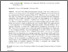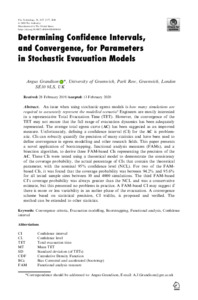# Determining confidence intervals, and convergence, for parameters in stochastic evacuation models

(2020) Determining confidence intervals, and convergence, for parameters in stochastic evacuation models. Fire Technology, 56. pp. 2137-2177. ISSN 0015-2684 (Print), 1572-8099 (Online) (doi:https://doi.org/10.1007/s10694-020-00968-0)Preview
PDF (Publisher's PDF - Open Access)
27065 GRANDISON_Determining_Confidence_Intervals_(OA)_2020.pdf - Published VersionPreview
PDF (Author's Accepted Manuscript)
27065 GRANDISON_Determining_Confidence_Intervals_And_Convergence_For_Parameters_In_Stochastic_Models_(AAM)_2020.pdf - Accepted Version

## Abstract

An issue when using stochastic egress models is how many simulations are required to accurately represent the modelled scenario? Engineers are mostly interested in a representative Total Evacuation Time (TET). However, the convergence of the TET may not ensure that the full range of evacuation dynamics has been adequately represented. The average total egress curve (AC) has been suggested as an improved measure. Unfortunately, defining a confidence interval (CI) for the AC is problematic. CIs can robustly quantify the precision of many statistics and have been used to define convergence in egress modelling and other research fields. This paper presents a novel application of bootstrapping, functional analysis measures (FAMs), and a bisection algorithm, to derive three FAM-based CIs representing the precision of the AC. These CIs were tested using a theoretical model to demonstrate the consistency of the coverage probability, the actual percentage of CIs that contain the theoretical parameter, with the nominal 95% confidence level (NCL). For two of the FAM-based CIs, it was found that the coverage probability was between 94.2% and 95.6% for all tested sample sizes between 10 to 4000 simulations. The third FAM-based CI’s coverage probability was always greater than the NCL and was a conservative estimate, but this presented no problems in practice. A FAM-based CI may suggest if there is more or less variability in an earlier phase of the evacuation. A convergence scheme based on statistical precision, CI widths, is proposed and verified. The method can be extended to other statistics.View Item